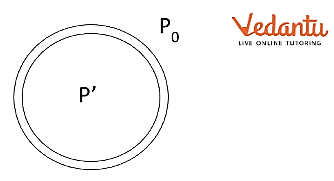Courses
Courses for Kids
Free study material
Free LIVE classes
More

# Excess Pressure inside a Liquid Drop for JEE## What are the Forces on a Liquid Drop?

Last updated date: 24th Mar 2023
Total views: 67.2k
Views today: 0.45k

You might have observed in your daily life and wondered that the free surface of water or any liquid becomes curved when it is in contact with a solid. This happens because of a phenomenon called surface tension. It occurs because of attraction between the molecules of the liquid. Generally, these attractive forces tend to cancel out each other between molecules. But, for the molecules on the surface, these forces don’t cancel out which results in surface tension.

Surface tension exists for every surface of a liquid and this force gives rise to an energy called surface energy. So, every liquid surface possesses some surface energy of its own. Here dominates a well-established fact that every system in nature tends to be in the lowest energy state. So a liquid surface tends to have the least surface energy which directly means the least surface area. So, the liquid drops are spherical. These spherical liquid drops have surface tension. This very surface tension generates an excess pressure inside the drop. In this article, we shall be discussing this excess pressure inside a liquid drop and bubble

## Excess Pressure inside a Liquid Drop

When there is a curved surface of a liquid, every molecule on the surface will experience a force that is along the tangent to that surface. When these force vectors are added, the horizontal components cancel out each other and the vertical components add up. So, the resultant force is normal to the surface. The pressure on the concave side of the curve will be greater than the pressure on the convex side because the pressure on the concave side must balance out the pressure on the convex side and the net inward force generated due to surface tension as well.

## Excess Pressure inside a Liquid Drop DerivationPressure on a Liquid Drop

Let us derive an expression for excess pressure inside a liquid drop. A spherical drop is shown in the figure above. Let $P$ be pressure inside the drop and ${{P}_{0}}$ be the outside pressure. Considering that the drop is spherical in shape, the inside pressure $P$ will be greater than the outside pressure ${{P}_{0}}$. So, the excess pressure inside the liquid drop will be $P\text{ }\text{ }{{P}_{0}}$.

Due to that pressure, let us assume the radius of drop increases from $r$ to $r+\Delta r$. Note that $\Delta r<<<r$ so, the pressure inside the drop almost remains the same.

Initial surface area of the drop ${{A}_{1}}=4\pi {{r}^{2}}$

Final surface area of the drop ${{A}_{2}}=4\pi {{\left( r+\Delta r \right)}^{2}}$

Expanding, ${{A}_{2}}=4\pi \left( {{r}^{2}}+2r\Delta r+\Delta {{r}^{2}} \right)$

We can neglect $\Delta {{r}^{2}}$ as it is very small as compared to the rest.

So, ${{A}_{2}}=4\pi {{r}^{2}}+8\pi r\Delta r$

Now, $dA={{A}_{2}}-{{A}_{1}}=8\pi r\Delta r$

Work done in increasing the surface area is the energy that will be converted to surface energy.

So, $dW=TdA=T\left( 8\pi r\Delta r \right)$     … (1)

We know that work done also equals to the product of force $F$ which caused an increase in surface area with the displacement $\Delta r$ of the surface

So, $dW=F\Delta r$                           … (2)

The excess force is given by, (excess pressure  $X$ surface area)

So, $F=\left( P'-{{P}_{0}} \right)4\pi {{r}^{2}}$              …  (3)

Equating (1) and (2),

$T\left( 8\pi r\Delta r \right)=F\Delta r$

$T\left( 8\pi r\Delta r \right)=\left( P'-{{P}_{0}} \right)4\pi {{r}^{2}}\Delta r$

$P'-{{P}_{0}}=\frac{2T}{R}$

This is the expression for excess pressure inside a liquid drop. We can use the above expression to find the excess pressure inside a liquid drop

## Excess Pressure inside a Soap Bubble

Unlike a liquid drop, a soap bubble has two surfaces with one being on the outside of the bubble and one inside the bubble. The reason behind the consideration of two surfaces in a soap bubble is that, unlike a liquid drop, there is air trapped inside the bubble, creating surface tension on two surfaces. As there are two surfaces, the excess pressure will also be doubled as the surface area is doubled. So, for a soap bubble, excess pressure $P\text{ } - \text{ }{{P}_{0}}=\text{ }2\text{ }X\text{ }\left( \text{ }2T/R \right)$.

## Conclusion

The excess pressure generated due to surface tension is a phenomenon responsible for many characteristics of a liquid. This excess pressure is responsible for the size of soap bubbles. If you have noticed, it is difficult to blow small bubbles or it is easier to inflate a balloon when it is already filled. This is because excess pressure is inversely proportional to the radius. So, the lesser the radius, the more will be the surface pressure.

## FAQs on Excess Pressure inside a Liquid Drop for JEE

1. Why is the inside pressure of a water droplet higher than the outside pressure?

The inside pressure of a water droplet is higher because the molecules of water at the surface create a net inward force on a droplet due to surface tension. So, pressure inside must be enough to balance the outside pressure and this net inward force. For this very reason, the inside pressure of a water droplet is higher than the outside pressure.

2. Why are liquid droplets spherical?

Liquid droplets are spherical to keep the surface energy minimum. Every surface of a liquid has surface energy because of surface tension. As per the law of nature, every system tends to be in the state of least energy. So, liquid tends to have the least surface energy, which can only be acquired in the case of the least surface area. For a given volume, a sphere will have the least surface area. This is the reason liquid drops are spherical.